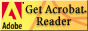Stankov's Universal Law Press

- Internet Publishing 2000 -

presents the following books by the discoverer of the

Universal Law of Nature,

Dr. Georgi Stankov, to download from this homepage:

 The Universal Law. The General Theory of Physics and Cosmology. Volume II (concise version), English, 290 pages, first published in 2000. 1,18 MB as PDF The General Theory of Biological Regulation. The Universal Law in Bioscience and Medicine. Volume III, English, 377 pages, first published in 2000. 1,18 MB as PDF Das Universalgesetz im Spiegelbild der Philosophie, Volume IV, German, 137 pages. 415 KB as PDF Die moderne Wirtschaftstheorie aus der Sicht des Universalgesetzes. Lectures, 84 pages. 204 KB as PDF Universalnijat zakon. Obshta teorija po fizika i kosmologija (The Universal Law. The General Theory of Physics and Cosmology). Volume II, full version with exercises, Bulgarian, 471 pages, first published in 1999.Универсалният закон. Обща теория по физика и космология. Том II, пълно издание, 471 стр., първа публикация 1999 г. 2,35 MB as PDF Universalnijat zakon. Kratko vavedenie v obshtata teorija na naukite i nejnoto otrazenie varhu obshtestvoto (The Universal Law. A Short Introduction Into the General Theory of Sciences and its Consequences for the Society). Bulgarian, 150 pages, 2nd edition in 1999. Универсалният закон. Кратко въведение в общата теория на науките и нейното отражение върху обществото, Bulgarian, 150 стр., второ издание 1999 г. 586 KB as PDF Universalnii (Vseobshchii) zakon. Kratkoe vvedenie v obshchuiu teoriiu nauki i vliijanie eio na obshtestvo (The Universal Law. A Short Introduction Into the General Theory of Sciences and its Consequences for the Society). Russian, 139 pages, first edition in 2000. Универсальный (Всеобщий) закон. Краткое введение в общую теорию науки и влияние ее на общество, Russian, 139 стр., первое издание 2000. 635 KB as PDF

Get Adobe Acrobat ReaderDas Universalgesetz, Volume I, German, 679 pages, 1997 can be ordered under:

Fax: (D)-89-9033446

[View my guestbook]

ABSTRACT

In 1995, I discovered the Universal Law of nature (the Law); I showed that all physical laws and their applications can be derived from this one law within mathematical formalism, and explained it epistemologically. This has led to the development of a unified theory of physics and cosmology, which is an axiomatization (axiomatics) of physics and mathematics. Thus physics is applied mathematics. The major results of this theory are: all terms in physics can be axiomatically derived from the primary term - energy = space-time (primary axiom). Energy (space-time) is closed, infinite, continuous, inhomogeneous (discrete), and constant; it is in a state of permanent energy exchange. The continuum (the set of all numbers) is equivalent to the primary term. The new axiomatics can be empirically verified. Thus the validity of mathematics as challenged by Godel’s theorem can be proven in the real word (proof of existence). This eliminates the continuum hypothesis and the foundation crisis of mathematics. The Universal Law describes space-time in terms of mathematics. The universal equation is E = EAf, where E is energy exchange, EA is a specific constant amount (quantum) of exchanged energy, called „action potential“, and f = E/EA is called „absolute time“. It is a dimensionless quotient. The Universal Law is a „law of energy“. Energy (space-time) is the only real thing. All physical quantities such as mass, charge, force, and momentum are abstract subsets of space-time that are defined within mathematics (objects of thought). They are dimensionless numbers that belong to the continuum. Since they contain space-time as an element (U-subsets), they can be derived in an axiomatic manner from the primary term. For instance, mass is a synonym for an energy (space-time) relationship and charge is a synonym for area, that is, the SI unit 1 coulomb is equivalent to 1 m2. This leads to the unification of physics and cosmology on the basis of mathematical formalism. This new physical and mathematical axiomatics also integrates gravitation with the other forces.